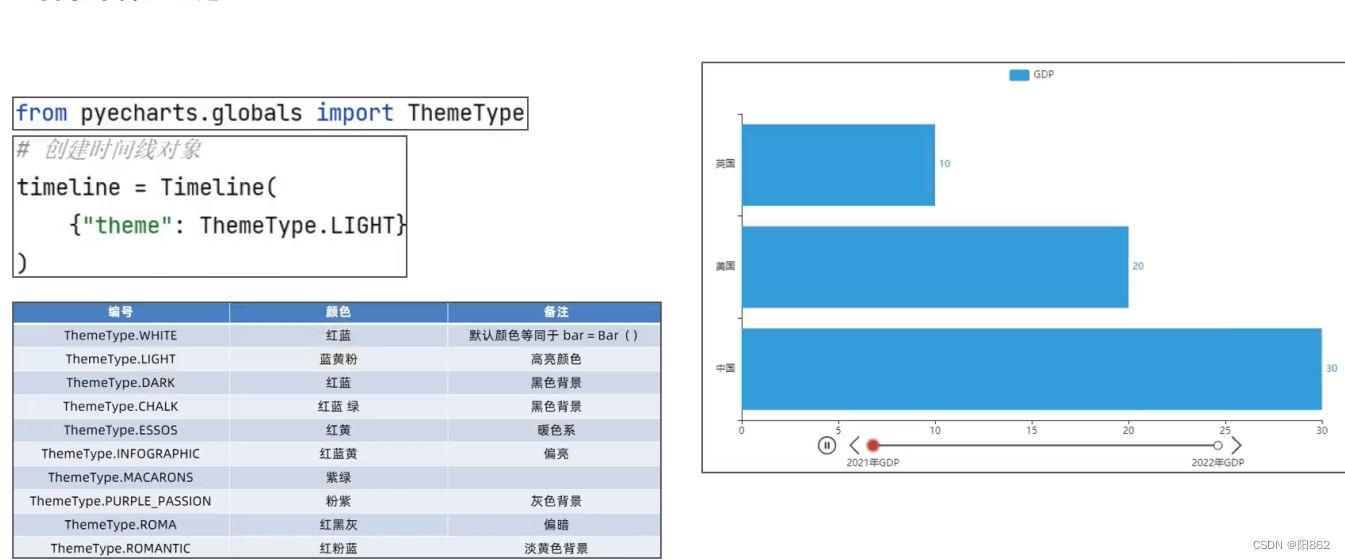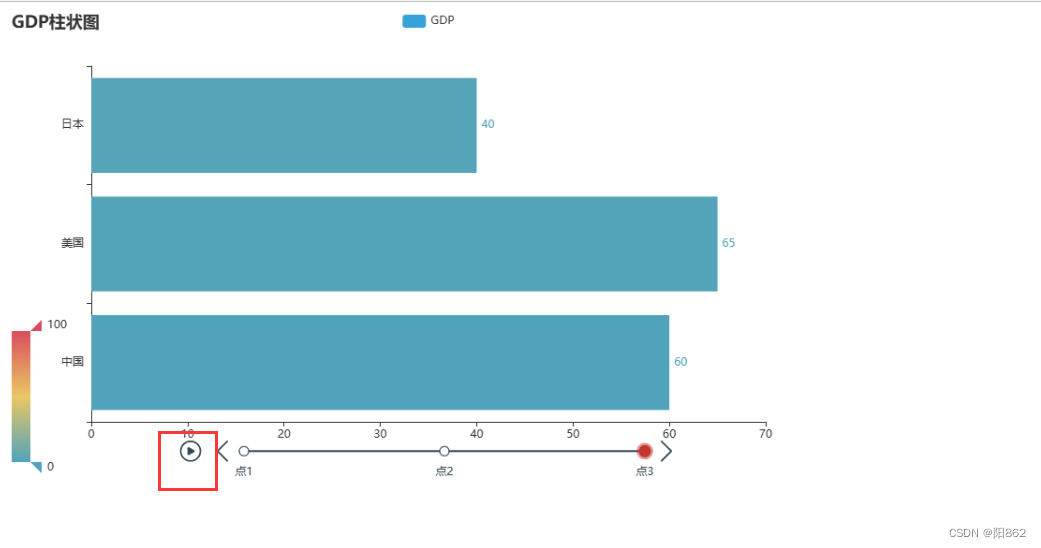# Python实现动态柱状图的绘制

2022-12-29 172阅读

## ?=一.基础柱状图`from pyecharts.charTS import Bar from pyecharts.optiONs import * #构建柱状图对象 bar=Bar() #添加x轴对象 bar.add_xaxis(["中国","美国","日本"]) #添加y轴对象 bar.add_yaxis("GDP",[40,50,30]) #设置全局选项 bar.set_global_opts( title_opts=TitleOpts(),#加名称 visualMap_opts=VisualmapOpts(#加范围显示 is_show=True ) ) #绘图 bar.render("基础柱状图.html")``#添加y轴对象 bar.add_yaxis("GDP",[40,50,30],Label_opts=LabelOpts(position="right")) #反转x轴y轴 bar.reversal_axis()`## 二.基础时间线柱状图`from pyecharts.charts import Bar,timeline from pyecharts.options import * from pyecharts.globals import Themetype #构建柱状图对象 bar1=Bar() #添加x轴对象 bar1.add_xaxis(["中国","美国","日本"]) #添加y轴对象 bar1.add_yaxis("GDP",[40,50,30],label_opts=LabelOpts(position="right")) #反转x轴y轴 bar1.reversal_axis() #设置全局选项 bar1.set_global_opts( title_opts=TitleOpts(),#加名称 visualmap_opts=VisualMapOpts(#加范围显示 is_show=True ) )     #构建柱状图对象 bar2=Bar() #添加x轴对象 bar2.add_xaxis(["中国","美国","日本"]) #添加y轴对象 bar2.add_yaxis("GDP",[50,60,45],label_opts=LabelOpts(position="right")) #反转x轴y轴 bar2.reversal_axis() #设置全局选项 bar2.set_global_opts( title_opts=TitleOpts(),#加名称 visualmap_opts=VisualMapOpts(#加范围显示 is_show=True ) )       #构建柱状图对象 bar3=Bar() #添加x轴对象 bar3.add_xaxis(["中国","美国","日本"]) #添加y轴对象 bar3.add_yaxis("GDP",[60,65,40],label_opts=LabelOpts(position="right")) #反转x轴y轴 bar3.reversal_axis() #设置全局选项 bar3.set_global_opts( title_opts=TitleOpts(),#加名称 visualmap_opts=VisualMapOpts(#加范围显示 is_show=True ) )     #构建时间线对象 timeline=Timeline({"theme":ThemeType.LIGHT}) #在时间线上添加柱状图对象 timeline.add(bar1,"点1") timeline.add(bar2,"点2") timeline.add(bar3,"点3")     #绘图是用时间线对象绘图，而不是用 bar了 timeline.render("基础时间线柱状图.html")``#自动播放设置 timeline.add_schema( play_interval=1000,#这里是放下一张图的时间间隔，毫秒为单位 is_timeline_show=True,#是否显示时间线 is_auto_play=True,#是否自动播放 is_loop_play=True#是否循环播放 )   #绘图是用时间线对象绘图，而不是用 bar了 timeline.render("基础时间线柱状图.html")`## 三.GDP动态柱状图绘制

1.了解列表的sort方法并配合Lambda匿名函数完成列表排序

`my_list=[["a",12],["b",4],["c",45]] def choose_sort_key(element): return element   my_list.sort(key=choose_sort_key,reverse=True) print(my_list)   my_list.sort(key=lambda element:element,reverse=False) print(my_list)`2.完成图表所需数据`#将数据转为字典存储，格式为： #{ 年份:[[国家, gdp]，[国家, gdp]，...... ]，年份:[[国家,gdp]，[国家, gdp]，.....], ...... }，比如： #{ 1960:[[美国, 123]，[中国, 231]，...... ]，1961:[[美国,124]，[中国, 234]，.....], ...... } #先定义一个字典 data_dict={} for line in data_lines: year=int(line.split(","))#年份 country=line.split(",")#省份 GDP=float(line.split(","))#因为美国的GDP是科学计数法，所以用float强制转回来 #如何判断字典里面有没有制定的key呢？ try: data_DIct[year].append([country,GDP]) except KeyError: data_dict[year]=[] data_dict[year].append([country,GDP])   print(data_dict)`3.完成GDP动态图表绘制`from pyecharts.charts import Bar,Timeline from pyecharts.options import *   #读取文件 f=open("D:/1960-2019全球GDP数据.csv","r",encoding="ANSI") data_lines=f.readlines() #关闭文件 f.Close() #删除第一行数据 data_lines.pop(0)   #将数据转为字典存储，格式为： #{ 年份:[[国家, gdp]，[国家, gdp]，...... ]，年份:[[国家,gdp]，[国家, gdp]，.....], ...... }，比如： #{ 1960:[[美国, 123]，[中国, 231]，...... ]，1961:[[美国,124]，[中国, 234]，.....], ...... } #先定义一个字典 data_dict={} for line in data_lines: year=int(line.split(","))#年份 country=line.split(",")#省份 GDP=float(line.split(","))#因为美国的GDP是科学计数法，所以用float强制转回来 #如何判断字典里面有没有制定的key呢？ try: data_dict[year].append([country,GDP]) except KeyError: data_dict[year]=[] data_dict[year].append([country,GDP])   #排序年份 sorted_year_list=sorted(data_dict.keys()) timeline = Timeline()#创建时间线对象 for year in sorted_year_list: data_dict[year].sort(key=lambda element:element,reverse=True) #取出本年份前八名的国家 year_data=data_dict[year][:8] x_data=[] y_data=[] for country_gdp in year_data: x_data.append(country_gdp)#x轴添加国家 y_data.append(country_gdp/100000000)#y轴添加GDP数据,单位为亿元   #构建柱状图 bar=Bar() bar.add_xaxis(x_data) bar.add_yaxis("GDP亿元",y_data,label_opts=LabelOpts(position="right")) #反转x轴，y轴 bar.reversal_axis()   #创建时间线对象   timeline.add(bar,str(year))   #设置时间为自动播放 timeline.add_schema( play_interval=1000,#时间间隔 is_timeline_show=True,#是否显示时间 is_loop_play=True,#是否循环 is_auto_play=True#是否自动播放 ) #绘图 timeline.render("1960——2019全球GDP前八国家.html")``#构建柱状图 bar=Bar() x_data.reverse() y_data.reverse() bar.add_xaxis(x_data) bar.add_yaxis("GDP亿元",y_data,label_opts=LabelOpts(position="right"))``from pyecharts.globals import ThemeType   timeline = Timeline({"theme":ThemeType.LIGHT})#创建时间线对象``#反转x轴，y轴 bar.reversal_axis() #设置每一年的图表标题 bar.set_global_opts( title_opts=TitleOpts(title=f"{year}年全球GDP前八数据") )`## 四.完整代码

`from pyecharts.charts import Bar,Timeline from pyecharts.options import * from pyecharts.globals import ThemeType   #读取文件 f=open("D:/1960-2019全球GDP数据.CSV","r",encoding="ANSI") data_lines=f.readlines() #关闭文件 f.close() #删除第一行数据 data_lines.pop(0)   #将数据转为字典存储，格式为： #{ 年份:[[国家, gdp]，[国家, gdp]，...... ]，年份:[[国家,gdp]，[国家, gdp]，.....], ...... }，比如： #{ 1960:[[美国, 123]，[中国, 231]，...... ]，1961:[[美国,124]，[中国, 234]，.....], ...... } #先定义一个字典 data_dict={} for line in data_lines: year=int(line.split(","))#年份 country=line.split(",")#省份 GDP=float(line.split(","))#因为美国的GDP是科学计数法，所以用float强制转回来 #如何判断字典里面有没有制定的key呢？ try: data_dict[year].append([country,GDP]) except KeyError: data_dict[year]=[] data_dict[year].append([country,GDP])   #排序年份 sorted_year_list=sorted(data_dict.keys()) timeline = Timeline({"theme":ThemeType.LIGHT})#创建时间线对象 for year in sorted_year_list: data_dict[year].sort(key=lambda element:element,reverse=True) #取出本年份前八名的国家 year_data=data_dict[year][:8] x_data=[] y_data=[] for country_gdp in year_data: x_data.append(country_gdp)#x轴添加国家 y_data.append(country_gdp/100000000)#y轴添加GDP数据,单位为亿元   #构建柱状图 bar=Bar() x_data.reverse() y_data.reverse() bar.add_xaxis(x_data) bar.add_yaxis("GDP亿元",y_data,label_opts=LabelOpts(position="right")) #反转x轴，y轴 bar.reversal_axis() #设置每一年的图表标题 bar.set_global_opts( title_opts=TitleOpts(title=f"{year}年全球GDP前八数据") ) #创建时间线对象   timeline.add(bar,str(year))   #设置时间为自动播放 timeline.add_schema( play_interval=1000,#时间间隔 is_timeline_show=True,#是否显示时间 is_loop_play=True,#是否循环 is_auto_play=True#是否自动播放 ) #绘图 timeline.render("1960——2019全球GDP前八国家.html")`

`本站提供的资源，都来自网络，版权争议与本站无关，所有内容及软件的文章仅限用于学习和研究目的。不得将上述内容用于商业或者非法用途，否则，一切后果请用户自负，我们不保证内容的长久可用性，通过使用本站内容随之而来的风险与本站无关，您必须在下载后的24个小时之内，从您的电脑/手机中彻底删除上述内容。如果您喜欢该程序，请支持正版软件，购买注册，得到更好的正版服务。侵删请致信E-mail：Goliszhou@gmail.com`
\$